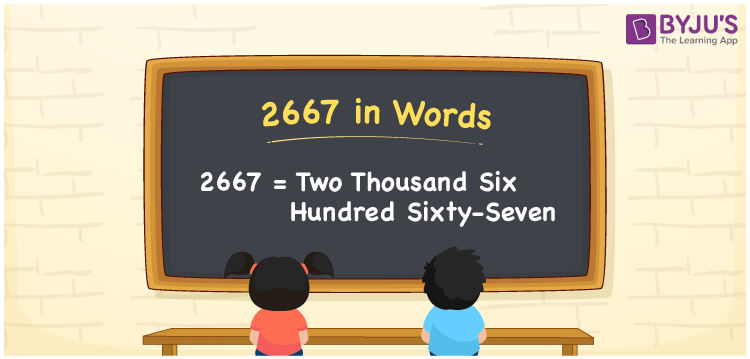# 2667 in Words

The number name of 2667 is Two Thousand Six Hundred Sixty-Seven. For example, Madhu purchased a dress worth Rs. 2667, then you can say, “Madhu purchased a dress worth Rupees Two Thousand Six Hundred Sixty-Seven”. 2667 is a cardinal number since it shows a specific amount. Writing numerical names by finding the position of each digit in the given number enhances students’ understanding capacity about numbers in words. Let us discuss how to convert the number 2667 using a place value system in a systematic manner.

 2667 in Words Two Thousand Six Hundred Sixty-Seven Two Thousand Six Hundred Sixty-Seven in numerical form 2667

## 2667 in English Words

In the education field, the most commonly used language is English. Hence, we generally prefer to write numbers in words using the letters of the English alphabet. Therefore, we can spell the number 2667 in words as Two Thousand Six Hundred Sixty-Seven.## How to Write 2667 in Words?

Let us learn how to write the numerical number for 2667 using a place value chart. First, we need to prepare a table of 4 columns as 2667 is a four-digit number. Let’s have a look at the place value chart for the number 2667 and its expanded form in the below table.

 Thousands Hundreds Tens Ones 2 6 6 7

Hence, we can write the expanded form as:

2 x Thousand + 6 x Hundred + 6 x Ten + 7 x One

= 2 x 1000 + 6 x 100 + 6 x 10 + 7 x 1

= 2000 + 600 + 60 + 7

= 2667

= Two Thousand Six Hundred Sixty-Seven

Therefore, 2667 in words is written as Two Thousand Six Hundred Sixty-Seven

Interesting way of writing 2667 in words

2 = Two

26 = Twenty-Six

266 = Two Hundred and Sixty-Six

2667 = Two Thousand Six Hundred Sixty-Seven

Thus, the word form of the number 2667 is Two Thousand Six Hundred Sixty-Seven

2667 is a natural number that is the successor of 2666 and the predecessor of 2668

• 2667 in words – Two Thousand Six Hundred Sixty-Seven
• Is 2667 an odd number? – Yes
• Is 2667 an even number? – No
• Is 2667 a perfect square number? – No
• Is 2667 a perfect cube number? – No
• Is 2667 a prime number? – No
• Is 2667 a composite number? – Yes

## Frequently Asked Questions on 2667 in Words

Q1

### How do to write 2667 in words?

We write 2667 in words as Two Thousand Six Hundred Sixty-Seven.
Q2

### Simplify 1000 + 1667, and express in words.

Simplifying 1000 + 1667, we get 2667. Therefore, the number 2667 in words is Two Thousand Six Hundred Sixty-Seven.
Q3

### Write Two Thousand Six Hundred Sixty-Seven in numbers.

Two Thousand Six Hundred Sixty-Seven in numbers is 2667.